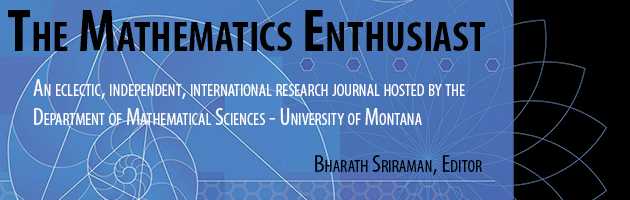•
•16

1-3

#### Abstract

In calculus-based statistics courses, the expected value of a random variable (EVORV) is discussed in relation to underlying mathematical notions. This study examines students’ understanding of the mathematical notions of EVORV in connection with its semiotic and lexical representations. It also assesses students’ computational competency revolving around EVORV. We collected qualitative data via surveys and interviews from eight students enrolled in a calculus-based university statistics course. The results suggest that while the students in general had the computational accuracy to correctly calculate EVORV, they struggled to understand the notion, and in particular to make sense of the term “random” in “random variable” and the symbol E(𝑋) in the mathematical context. The study provides a basis for understanding potential challenges to students’ learning of EVORV and other related statistics topics and how such challenges may emerge from the semiotic and lexical ambiguities inherent in terms and symbols used in statistics.

231

252

COinS